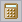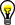# Calculating Formulas in the Calculator

Edition Date: July 2011

Part Number: 370859J-01

»View Product InfoDownload Help (Windows Only)

Use the Calculator to calculate data with your own formulas. In formulas you can combine numeric channels, waveform channels, and time channels and generate new channels. You use the text operations for calculating with text channels. You can calculate single values, save values in variables, and request variable contents.To open the Calculator, click the DIAdem ANALYSIS toolbar button shown here.

## Entering Formulas

Use the keyboard and the Calculator keys to enter your formula in the Enter field. The Calculator displays the calculated result in the result box.

A formula consists of the assignment target, the assignment operator, and the calculation statement:

Assignment target = Calculation statement

You usually enter a channel as the assignment target. However, you also can calculate a single value and assign the single value to a variable. In the calculation statement you use operations on channels or variables. The tabs at the bottom left of the Calculator have numeric operations such as the sine function, Boolean operations such as AND, and text operations such as text length.

For example, if you want to calculate the sine values of a channel, double-click sin(arg) on the Numeric Operations tab to enter the sine function in the Enter field. The cursor in the Enter field is automatically positioned in the argument section of the sine function, so you can double-click the channel you want in the Channels tab to paste the channel into your formula.

In the Calculator, always use a decimal point as the decimal separator and enclose strings in quotation marks ("...").Note  To use the operations, channels, and variables tabs in the Calculator, click the Extended button.

### Calculating with Channels

For example, to divide the Input channel by the Reference channel from the first channel group, enter the following formula in the Calculator Enter field:

Ch("/Result") = Ch("/Input")/Ch("/Reference")

DIAdem divides each value of the Input channel by the corresponding value of the Reference channel. If the channels are different lengths, the result channel is the same length as the shorter channel.

DIAdem stores the result in the Result channel in the first channel group. If the Result channel already exists in the first channel group, DIAdem overwrites the values. If you enter only the channel name without the group index, DIAdem stores the result channel in the default group of the Data Portal.

If you calculate with channels in the Calculator, the channels must have a unique reference. To use channels in calculations, use the Ch variable and enclose the channel reference in quotation marks. By default, DIAdem uses the group index and the channel name to create a unique channel reference. However, a channel reference can also consist of the group name or the group index combined with the channel name or the channel index. The group index is the position of the group in the Data Portal and the channel index is the position of the channel in the group.

If you know the names of the channel groups and of the channels of the data set, and if the name of the first group is Group1, enter the following formula:

Ch("Group1/Result") = Ch("Group1/Input")/Ch("Group1/Reference")

If you know the structure of a data set, you can combine the group index and the channel index. Then enter the last formula as follows:

Ch("/") = Ch("/")/Ch("/")Note  You can also use only the channel name for the channel reference. This channel reference is ambiguous if several channels in different groups of the Data Portal have the same name. DIAdem calculates with the first channel it finds with the specified name in the Data Portal.

### Calculating with Variables and Single Values

In the Calculator, you can store values in variables, use variables in calculation statements, and request variable values. The following examples show how to enter these three alternatives for the auxiliary variable R1 in the Enter field, for example:

You assign the result of the square root of 8.391 to the variable R1:

R1 = SQR(8.391)

Enter a question mark after the variable name to request the value. The Calculator displays the value in the result box:

R1?Tip  To calculate a formula without saving the result, enter only the calculation instruction in the Enter field and enter a question mark at the end. The Calculator then shows the result only in the result box.

You multiply each value of the Input channel from the first channel group by the R1 variable. DIAdem stores the result values in the Result channel of the default group:

Ch("Result") = Ch("/Input")*R1